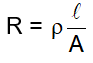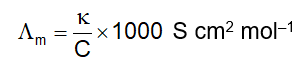# Chemistry formula for class 12 chapter- Electrochemistry

Sep 06, 2022, 16:45 IST

## Electrochemistry

The branch of chemistry which deals with the relationship between chemical energy and electrical energy and how one can be converted into another is known as electrochemistry.

### Electrolytic conduction

The flow of electric current through an electrolytic solution is called electrolytic conduction.

### Weak electrolytes

Those electrolytes which do not ionise completely in aqueous solution or in molten state are called weak electrolytes. e.g., H2CO3 (carbonic acid), H3BO3 (Boric acid), HCN (hydrocyanic acid), CH3COOH (acetic acid), CHCl2COOH (dichloroacetic acid), HgCl2 (Mercuric chloride), etc. They have small degree of ionisation.

### Strong electrolytes

Those electrolytes which dissociate completely into ions are called strong electrolytes, e.t., NaCl, HCl, NaOH etc.

### Degree of ionisation

It is the ratio of number of ions produced to the total number of molecules of electrolyte.

### Ohm’s law

The strength of current (I) flowing through a conductor is directly proportional to the potential difference (V) applied across the conductor and inversely proportional to resistance (R) of the conductor.

Mathematically, I = V/R

### Conductance

The ease with which current flows through a conductor is called its conductance. It is reciprocal of the resistance. C = 1/R. The unit of conductance is Siemens, S (i.e., 1S = 1 ohm–1 = 1Ω-1).

### Specific resistivity

If a solution is placed between two parallel electrodes having cross-sectional area ‘A’ and distance l apart, thenwhere ρ is resitivity.

### Specific conductivity (Electrolytic conductivity)

It is the reciprocal of specific resistivity of an electrolytic solution. It is denoted by Κ(kappa) and its unit is ohm–1 cm–1 or S cm–1. If A is expressed in m2, l in m and ‘R’ in ohm (Ω) , then unit of Κ is ohm–1 or S m–1.

Κ = C x l/A, Where C is conductance of solution

### Conductivity

The conductivity of solution of different electrolytes in the same solvent and at a given temperature difference due to the difference in charge and size of the ions in which they dissociate, the concentration of ions or ease with which the ions move under potential gradient.

### Molar Conductivity

It is defined as the conducting power of al the ions produced by one gram mole of an electrolyte in a solution. It is denoted by Λm.Where 'Κ' is electrolytic conductivity of solution and ‘C’ is concentration of the solution expressed in mol L–1 (or mol dm–3). The unit of is ohm–1 cm2 mol–1 or S cm2 mol–1.

1 S m2 mol–1 = 104 S cm2 mol1 Or 1 S cm2 mol–1 = 10–4 S m2 mol–1

Do find the detail NCERT Solutions for class 12 chemistry prepared by expert faculty of Physics Wallah. If any students need to take the online test to check their concepts or undertstanding then they can visit Quiz for Electrochemistry. Students can also get the CBSE Important Questions for class 12 Chemistry from here.

Download free pdf sheet which consist of formulas and important points of Electrochemistry from the link given below .